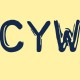I was at Jason Dyer‘s talk at the Tucson MEAD conference in January where he introduced me to these puzzles. The gist is: dotted lines indicate that circles should add to the desired result, while solid lines indicate that circles should multiply to the desired results. Here’s a worked-out example from Jason’s instructionsI find myself solving these semi-algebraically and checking my answers to see if they logically make sense. In the example above, I would start by asking myself “What do I need to multiply -3 by in order to get 6? Oh – the answer must be -2”. I know I’m correct because -3 + 1 = -2, and -3 * -2 = 6. I love these puzzles because they are self-checking and subtly emphasize checking your algebra work by validating that the arithmetic statement is actually true.

At this point, check out Jason’s other posts on these (specifically the example worksheets he’s done) and try a few yourself. You could even be daring and see the one that’s equivalent to factoring a cubic. Go ahead, the other stuff I have to say will only be meaningful if you understand the puzzles and appreciate them like I do.

Okay – so here’s a fun fact about me: I’m an amateur programmer. I’ve had this idea for a while and on this particularly lazy Sunday, I decided to put it in to action: I wrote several programs which generate these puzzles. They’re all websites that use HTML5 (in particular, the canvas element) and javascript to generate these puzzles. I say this because you’ll need an up-to-date browser to run these. Since I’m an amateur programmer, I don’t care too much about compatibility and all that other junk web programmers have to worry about, so I’ll just let you know that I’ve only tested it on Google Chrome on a Windows machine – no guarantees on anything else. With that said, here they are:

Update: I don’t think they work in Internet Explorer. Sorry…

Puzzle 1

Puzzle 2

Puzzle 3

Puzzle 4

Puzzle 5

Each puzzle will generate new numbers every time the page is loaded – so, if you want new numbers, just refresh the page. You can also generate the answers by pressing the ‘Generate Answer’ button. Lastly, if you press the ‘Generate Image’ button, an image of the canvas will appear below the buttons, allowing you to save the file to your computer and use in worksheets/presentations/etc. If you know what you’re looking for, you can also look through my code to see how I did all of it.

After the jump: what each of these puzzles is equivalent to and how I have an idea to use them in a Computer Science class I’m teaching this summer

So, here are the spoilers:

You can solve all the Puzzle 1’s by dividing the final number by the previous number, then subtracting the two to figure out the missing dotted line. Good algebra practice.

You can solve all the Puzzle 2’s by listing every factor-pair of the final number and seeing which pairs differ by the number on the far left. I used the word ‘factor-pair’ to mean any two numbers which multiply to give the final number – ie: a factor pair of 15 is (5, 3). Good factoring practice.

You can solve all Puzzle 3’s by listing every factor pair and finding the pair whose sum is the left number. Good factoring practice.

You can solve all Puzzle 4’s by subtracting the lower-left number from the far right-number, which is the number that goes in the top-center circle. Then subtract the top-center from the top-left to get the mid-center number, then subtract the mid-center number from the lower-left number to get the bottom-center number. That might be hard to describe in writing, but the key point is that the lower-left number + top-center number = far-right number, which lets me solve for the top-center number.

The Puzzle 5’s are equivalent to factoring the quadratic with the center numbers as coefficients. One algorithm for factoring (the AC Method) can be found here.

Here’s another reason I think these are cool: What I’ve essentially done in the lines above is describe an algorithm that you can use to solve these problems. One of the most challenging parts of teaching programming (for me, at least – but I’m still a novice programming teacher) is helping students to transition into algorithmic thinking – taking these things that make sense on a visual or trial-and-error level and finding a systematic way of making a computer do this. Things I’ve done in the past have been having students write algorithms for tying their shoes, brushing their teeth, making a PB&J sandwich – but, I’ve never had a good transition from these toy examples to a real programming example. I think these problems could help with the transition.

I’m teaching a Computer Science course this summer through the Johns Hopkins Center for Talented Youth program and I think I’m going to use these problems as a way to introduce the idea that some problems can be solved systematically and it is the challenge of the programmer to find the words or commands to solve these problems. Once my students figure this out, maybe I’ll have them write programs to solve these problems. I dunno – they’re all ideas right now. In the mean time, at least I can now generate as many of these puzzles as I want for use in my classroom next year.

From → Math

1.2.